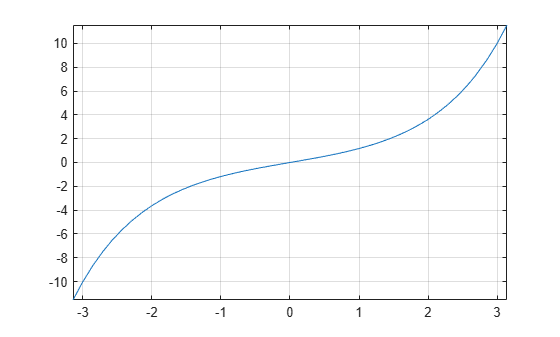# sinh

Symbolic hyperbolic sine function

## Syntax

``sinh(X)``

## Description

example

````sinh(X)` returns the hyperbolic sine function of `X`.```

## Examples

### Hyperbolic Sine Function for Numeric and Symbolic Arguments

Depending on its arguments, `sinh` returns floating-point or exact symbolic results.

Compute the hyperbolic sine function for these numbers. Because these numbers are not symbolic objects, `sinh` returns floating-point results.

`A = sinh([-2, -pi*i, pi*i/6, 5*pi*i/7, 3*pi*i/2])`
```A = -3.6269 + 0.0000i 0.0000 - 0.0000i 0.0000 + 0.5000i... 0.0000 + 0.7818i 0.0000 - 1.0000i```

Compute the hyperbolic sine function for the numbers converted to symbolic objects. For many symbolic (exact) numbers, `sinh` returns unresolved symbolic calls.

`symA = sinh(sym([-2, -pi*i, pi*i/6, 5*pi*i/7, 3*pi*i/2]))`
```symA = [ -sinh(2), 0, 1i/2, sinh((pi*2i)/7), -1i]```

Use `vpa` to approximate symbolic results with floating-point numbers:

`vpa(symA)`
```ans = [ -3.6268604078470187676682139828013,... 0,... 0.5i,... 0.78183148246802980870844452667406i,... -1.0i]```

### Plot Hyperbolic Sine Function

Plot the hyperbolic sine function on the interval from $-\pi$ to $\pi$.

```syms x fplot(sinh(x),[-pi pi]) grid on```### Handle Expressions Containing Hyperbolic Sine Function

Many functions, such as `diff`, `int`, `taylor`, and `rewrite`, can handle expressions containing `sinh`.

Find the first and second derivatives of the hyperbolic sine function:

```syms x diff(sinh(x), x) diff(sinh(x), x, x)```
```ans = cosh(x) ans = sinh(x)```

Find the indefinite integral of the hyperbolic sine function:

`int(sinh(x), x)`
```ans = cosh(x)```

Find the Taylor series expansion of `sinh(x)`:

`taylor(sinh(x), x)`
```ans = x^5/120 + x^3/6 + x```

Rewrite the hyperbolic sine function in terms of the exponential function:

`rewrite(sinh(x), 'exp')`
```ans = exp(x)/2 - exp(-x)/2```

## Input Arguments

collapse all

Input, specified as a symbolic number, variable, expression, or function, or as a vector or matrix of symbolic numbers, variables, expressions, or functions.

## Version History

Introduced before R2006a